# Angle BisectorSelection prerequisites: Either of the following:

Three points, with the vertex point selected second

The second selected point designates the vertex of the angle. For instance, to bisectABC, select points in the order: first A, then B, and finally C.

Two rays or segments with a common endpoint

You can optionally select the common endpoint as well. This makes it easy to use a selection rectangle to select the vertex and both sides of an angle.

This Construct menu command constructs a ray that bisects the minor angle formed by the three selected points or two selected straight objects.

 Selected Objects: Resulting Construction: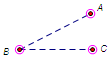Three points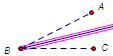Angle bisector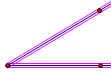Two rays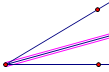Angle bisectorTwo segments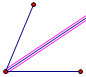Angle bisector